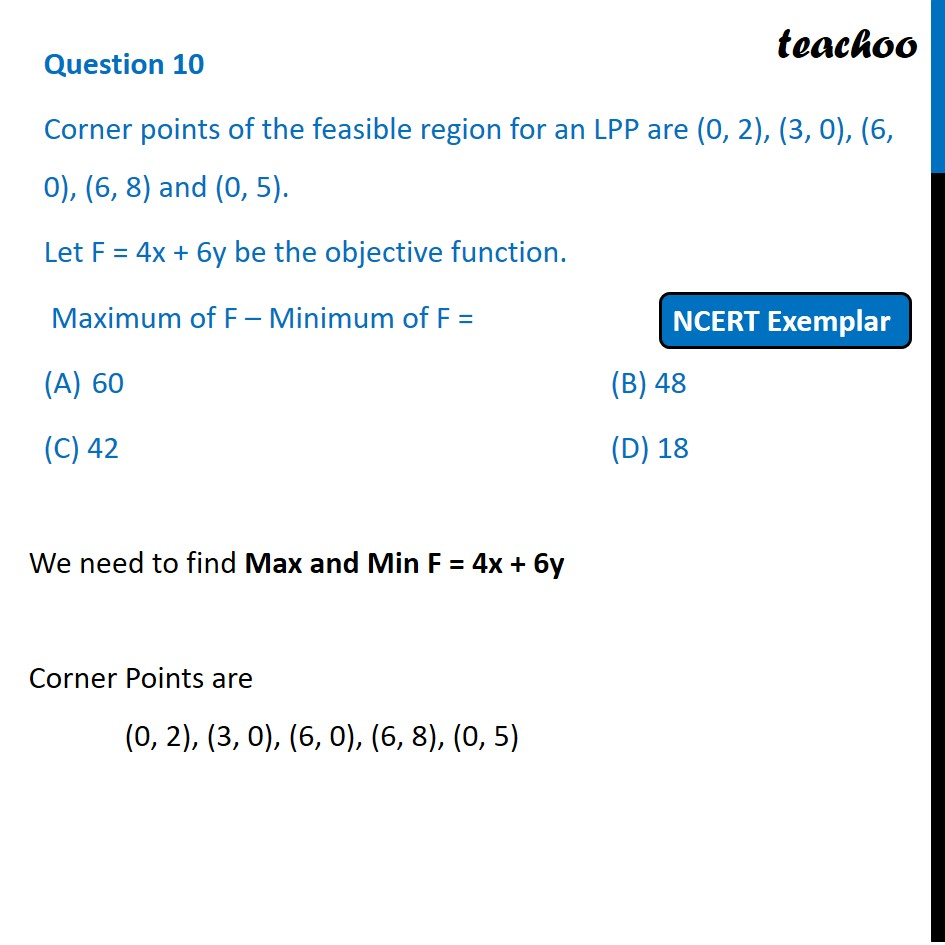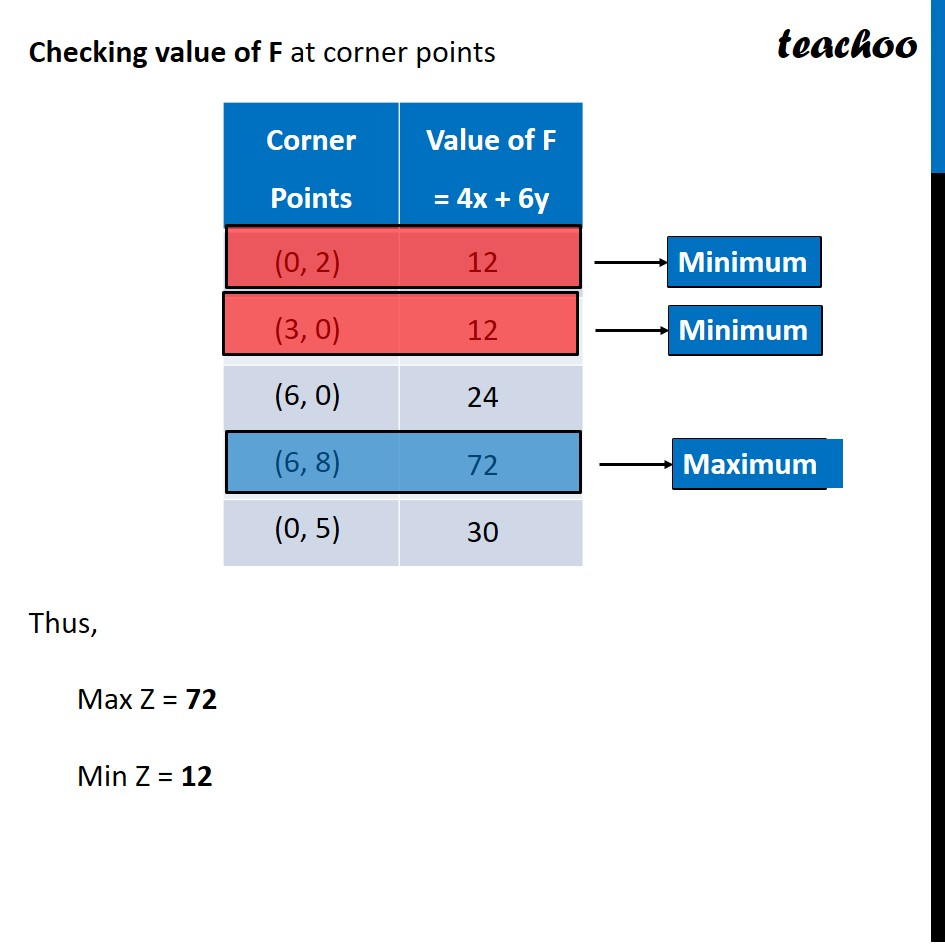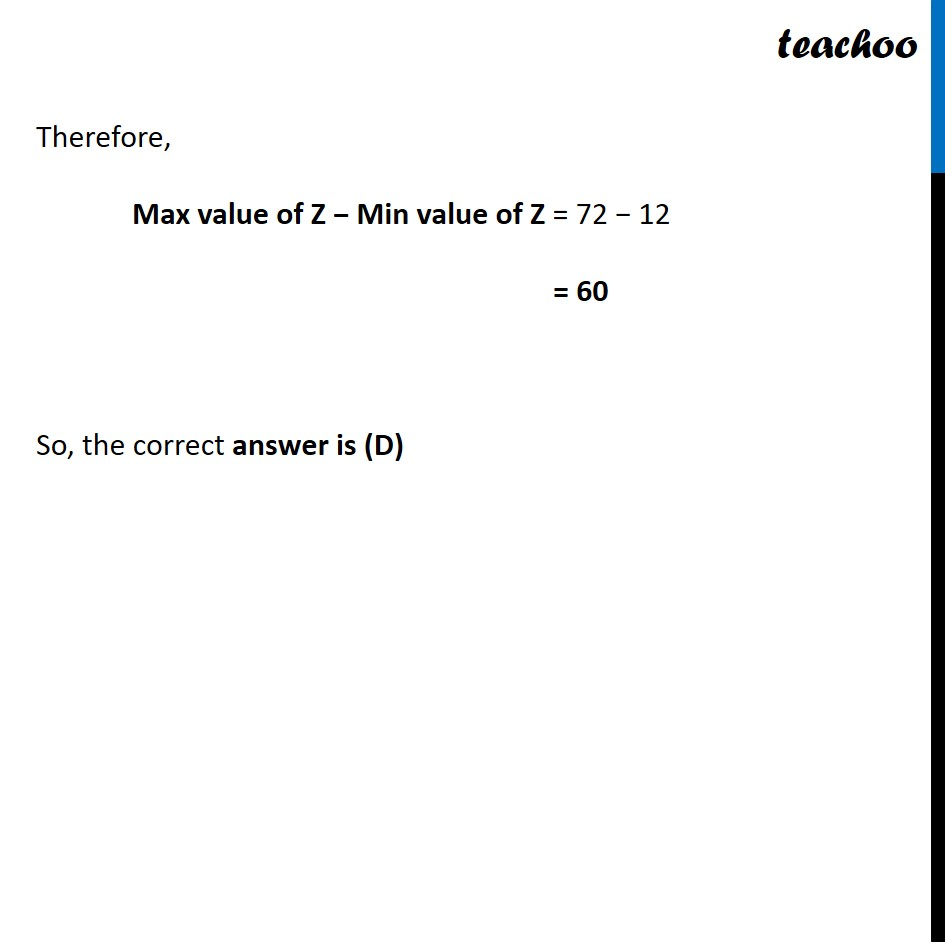NCERT Exemplar - MCQs

Chapter 12 Class 12 Linear Programming
Serial order wise

## (C) 42                         (D) 18

This question is similar to Question 38 Choice 2 - Sample Paper 2021 Class 12 - Linear ProgrammingLearn in your speed, with individual attention - Teachoo Maths 1-on-1 Class

### Transcript

Question 10 Corner points of the feasible region for an LPP are (0, 2), (3, 0), (6, 0), (6, 8) and (0, 5). Let F = 4x + 6y be the objective function. Maximum of F – Minimum of F = 60 (B) 48 (C) 42 (D) 18 We need to find Max and Min F = 4x + 6y Corner Points are (0, 2), (3, 0), (6, 0), (6, 8), (0, 5) Checking value of F at corner points Thus, Max Z = 72 Min Z = 12 Therefore, Max value of Z − Min value of Z = 72 − 12 = 60 So, the correct answer is (D)